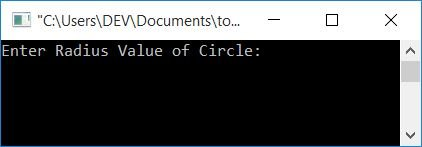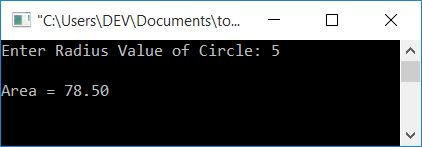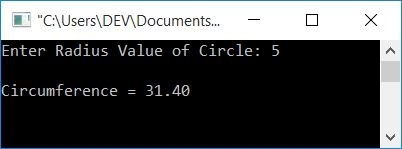# C program to calculate the area and circumference of a circle

In this article, you will learn and get code for calculating the area and circumference of a circle using C programs.

To calculate the area or circumference of any circle in C programming, you have to ask the user to enter the circle's radius, say r. To find an area using this radius, use 3.14*r*r. 2*3.14*r is used to calculate circumference. 3.14 is the value of π in this case.

## Calculate the area of the circle in C

The area of any circle can be calculated using the formula πr2. Here, r represents the radius of a circle, and the value of π is equal to 3.14. So the formula can be written as 3.14r2 or 3.14*r*r. Let's take a look at the program:

```#include<stdio.h>
#include<conio.h>
int main()
{
printf("Enter Radius Value of Circle: ");
printf("\nArea = %0.2f", area);
getch();
return 0;
}```

This program was written in the Code::Blocks IDE. Here is the first snapshot of the sample run:Now supply any number as the radius value of the circle, say 5, and press the ENTER key to see the output as shown in the second snapshot of the sample run:The format specifier, %0.2f, is used to print the value of area up to only two decimal places.

## Calculate the Area of a Circle Using a User-Defined Function in C

Let's create another program that does the same job but uses the function as shown here:

```#include<stdio.h>
#include<conio.h>
float findArea(float);
int main()
{
printf("Enter Radius Value of Circle: ");
printf("\nArea = %0.2f", area);
getch();
return 0;
}
{
}```

After compiling and executing the above program, you will get the same output as seen from the first program's output.

## Calculate the circumference of a circle in C

The circumference of a circle can be calculated using the formula 2πr, where the value of π is 3.14, and r is the radius of the circle. By adding the value of π, the formula becomes 2*3.14*r. Here is the program to find the circumference of a circle:

```#include<stdio.h>
#include<conio.h>
int main()
{
float r, circumf;
printf("Enter Radius Value of Circle: ");
scanf("%f", &r);
circumf = 2*3.14*r;
printf("\nCircumference = %0.2f", circumf);
getch();
return 0;
}```

Here is a sample run of the above program:## Using a C Function, calculate the circumference of a circle

Now let's create another program that does the same job as the previous program, but this time using functions.

```#include<stdio.h>
#include<conio.h>
float findCircumf(float);
int main()
{
float r, circumf;
printf("Enter Radius Value of Circle: ");
scanf("%f", &r);
circumf = findCircumf(r);
printf("\nCircumference = %0.2f", circumf);
getch();
return 0;
}
float findCircumf(float r)
{
return 2*3.14*r;
}```

This program produces the same output as seen in the previous program's output.

#### The same program in different languages

C Quiz

« Previous Program Next Program »